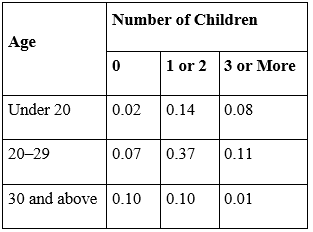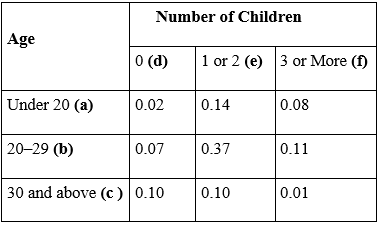# MA 121 Introduction to Statistics Coaching Session 2.1.1 #23

23) Hi this is Asmita! I am a Student Success Coach and will be explaining some practice problems from the MA121 Introduction to Statistics course. Today we will review an assigned problem from Unit 2. In the “Homework Assessment” you were asked to solve this problem:

The following two-way contingency table gives the breakdown of the population of married or previously married women beyond child-bearing age in a particular locale according to age at first marriage and number of children:A woman is selected at random. Find the probability of each of the following events.
a. The woman was in her twenties at her first marriage.
b. The woman was 20 or older at her first marriage.
c. The woman had no children.
d. The woman was in her twenties at her first marriage and had at least three children.

The first step in approaching this problem is to write sample space for the experiment and identify the probabilities for each outcome.
The experiment is the action of selecting a woman from the population of married or previously married women from a particular locale. We can label each outcome as a pair of letters, first of which represents age (a: under 20, b: 20-29, and c: 30 and above) and second represents number of children (d: no children, e: 1 or 2, and f: 3 or more).With all possible outcomes, the sample space can be written as
S = {ad, ae, af, bd, be, bf, cd, ce, cf}

From the given table,
Probability of a woman who is under 20 and has no children is P(ad) = 0.02
Probability of a woman who is under 20 and has 1 or 2 children is P(ae) = 0.14
Probability of a woman who is under 20 and has 3 or more children is P(af) = 0.08
Probability of a woman who is between ages of 20-29 and has no children is P(bd) = 0.07
Probability of a woman who is between ages of 20-29 and has 1 or 2 children is P(be) = 0.37
Probability of a woman who is between ages of 20-29 and has 3 or more children is P(bf) = 0.11
Probability of a woman who is of age 30 and above and has no children is P(cd) = 0.10
Probability of a woman who is of age 30 and above and has 1 or 2 children is P(ce) = 0.10
Probability of a woman who is of age 30 and above and has 3 or more children is P(cf) = 0.01

The concept that needs to be used is definition of “Probability of event.”

Steps to find probabilities are as follows:
1)Define the event
2)Write all possible outcomes for each event
3)Use definition of “Probability of an event”
4)Plug the values to find the required probability

a.
Step 1: Define the event:
Event T is the action of selecting the woman who was in her twenties at her first marriage.
This means that the woman was married between ages 20 – 29 and may have either ‘0’ children or ‘1-2’ children or ‘3 or more’ children.

Step 2: Write all possible outcomes in event T:
Outcomes bd,be, and bf from sample space considers women who were married between ages 20-29 (irrespective of number of children).
Therefore, T = {bd, be, bf}

Step3: Use definition of “Probability of an event”:
To find the probability of the event, we find the sum of probability of individual outcomes in the event. Therefore,
P(T) = P (bd) + P(be) + P (bf)

Step 4: Plug the values to find the required probability:
P(T) = P (bd)+ P(be) + P (bf) = 0.07 + 0.37 + 0.11 = 0.55

b.

Step 1: Define the event:
Event O is the action of selecting the woman who was 20 or older at her first marriage.
This means that the woman was married in between ages ‘20 – 29’ or in ages ‘30 or more’ and may have either ‘0’ children or ‘1-2’ children or ‘3 or more’ children.

Step 2: Write all possible outcomes in event O:
Outcomes bd,be,bf,cd,ce, and cf from the sample space considers women who were 20 or older (irrespective of whether they had children).
Therefore, O = {bd, be, bf, cd, ce, cf}

Step 3: Use definition of “Probability of an event”:
To find the probability of the event, we find the sum of probability of individual outcomes in the event. Therefore,
P(O) = P( bd) + P( be) + P(bf) + P(cd) + P(ce) + P(cf)

Step 4: Plug the values to find the required probability:
P(O) = P( bd ) + P(be) + P( bf ) + P( cd ) + P( ce ) + P( cf )
P(O) = 0.07 + 0.37 + 0.11 + 0.10 + 0.10 + 0.01
P(O) = 0.76

c.

Step 1: Define the event:
Event NC is the action of selecting a woman who had no children.
This means that the woman has 0 children and may be ‘under 20’ or ‘between ages 20-29’ or ‘30 or more.’

Step 2: Write all possible outcomes in event NC:
Outcomes ad,bd, and cd from the sample space considers women who had no children (irrespective of their age).
Therefore, NC = {ad, bd, cd}

Step 3: Use definition of “Probability of an event”:
To find the probability of the event, we find the sum of probability of individual outcomes in the event. Therefore,

Step 4 : Plug the values to find the required probability:
P(NC) = P(ad) + P( bd) + P(cd)
P(NC) = 0.02 + 0.07 + 0.10 = 0.19

d.
Step 1: Define the event:
Event TC is an action of selecting a woman was in her twenties at her first marriage and had at least three children.
This means the woman would be in between ages 20 - 29 and has 3 or more children at the same time.

Step 2: Find probability of event TC
Outcome in the sample space, which represents this situation is f .
Therefore, TC = {bf}

Step 3: Use the definition of “Probability of an event”:
To find the probability of the event, we find the sum of probability of individual outcomes in the event. Therefore,
P(TC) = P(bf)

Step 4: Plug the values to find the required probability:
As there is only one outcome in event TC, P(TC)= P(bf) = 0.11.

Conclusion:
Probabilities for each of given events are as follows:
a.The woman was in her twenties at her first marriage = 0.55
b.The woman was 20 or older at her first marriage = 0.76
c.The woman had no children = 0.19
d.The woman was in her twenties at her first marriage and had at least three children = 0.11

In addition to this, I am also attaching the pdf document of the practice problem for your ready reference.

Question 23.pdf (274.1 KB)

Please let me know if you have any question on this problem, or on this topic generally. I will be here in the forum for the next hour.

1 Like